# 12.5 Conic sections in polar coordinates  (Page 6/8)

 Page 6 / 8

$\frac{{x}^{2}}{9}-\frac{{y}^{2}}{16}=1$

$\frac{{\left(y-1\right)}^{2}}{49}-\frac{{\left(x+1\right)}^{2}}{4}=1$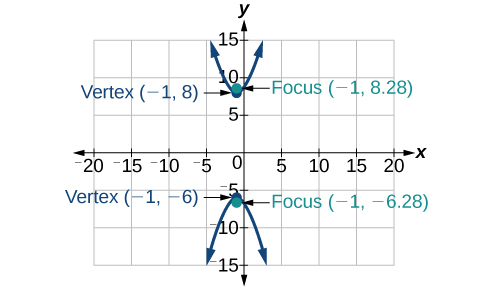${x}^{2}-4{y}^{2}+6x+32y-91=0$

$2{y}^{2}-{x}^{2}-12y-6=0$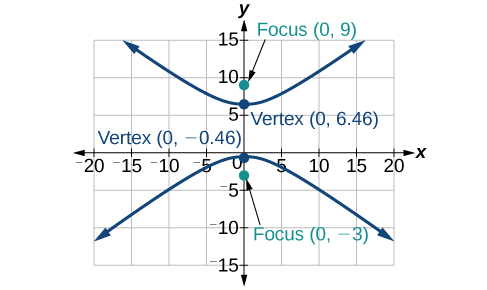For the following exercises, find the equation of the hyperbola.

Center at $\text{\hspace{0.17em}}\left(0,0\right),$ vertex at $\text{\hspace{0.17em}}\left(0,4\right),$ focus at $\text{\hspace{0.17em}}\left(0,-6\right)$

Foci at $\text{\hspace{0.17em}}\left(3,7\right)\text{\hspace{0.17em}}$ and $\text{\hspace{0.17em}}\left(7,7\right),$ vertex at $\text{\hspace{0.17em}}\left(6,7\right)$

$\frac{{\left(x-5\right)}^{2}}{1}-\frac{{\left(y-7\right)}^{2}}{3}=1$

## The Parabola

For the following exercises, write the equation of the parabola in standard form. Then give the vertex, focus, and directrix.

${y}^{2}=12x$

${\left(x+2\right)}^{2}=\frac{1}{2}\left(y-1\right)$

${\left(x+2\right)}^{2}=\frac{1}{2}\left(y-1\right);\text{\hspace{0.17em}}$ vertex: $\text{\hspace{0.17em}}\left(-2,1\right);\text{\hspace{0.17em}}$ focus: $\text{\hspace{0.17em}}\left(-2,\frac{9}{8}\right);\text{\hspace{0.17em}}$ directrix: $\text{\hspace{0.17em}}y=\frac{7}{8}$

${y}^{2}-6y-6x-3=0$

${x}^{2}+10x-y+23=0$

${\left(x+5\right)}^{2}=\left(y+2\right);\text{\hspace{0.17em}}$ vertex: $\text{\hspace{0.17em}}\left(-5,-2\right);\text{\hspace{0.17em}}$ focus: $\text{\hspace{0.17em}}\left(-5,-\frac{7}{4}\right);\text{\hspace{0.17em}}$ directrix: $\text{\hspace{0.17em}}y=-\frac{9}{4}$

For the following exercises, graph the parabola, labeling vertex, focus, and directrix.

${x}^{2}+4y=0$

${\left(y-1\right)}^{2}=\frac{1}{2}\left(x+3\right)$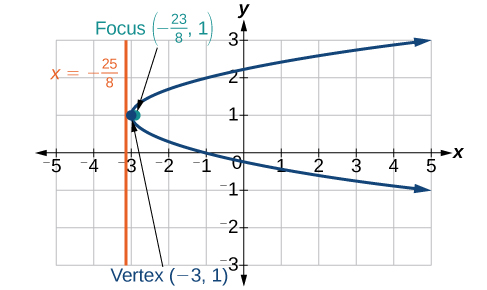${x}^{2}-8x-10y+46=0$

$2{y}^{2}+12y+6x+15=0$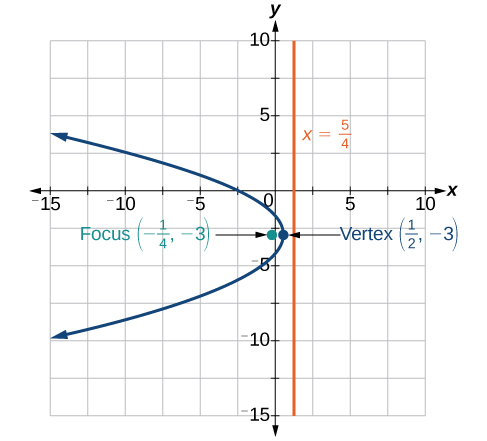For the following exercises, write the equation of the parabola using the given information.

Focus at $\text{\hspace{0.17em}}\left(-4,0\right);\text{\hspace{0.17em}}$ directrix is $\text{\hspace{0.17em}}x=4$

Focus at $\text{\hspace{0.17em}}\left(2,\frac{9}{8}\right);\text{\hspace{0.17em}}$ directrix is $\text{\hspace{0.17em}}y=\frac{7}{8}$

${\left(x-2\right)}^{2}=\left(\frac{1}{2}\right)\left(y-1\right)$

A cable TV receiving dish is the shape of a paraboloid of revolution. Find the location of the receiver, which is placed at the focus, if the dish is 5 feet across at its opening and 1.5 feet deep.

## Rotation of Axes

For the following exercises, determine which of the conic sections is represented.

$16{x}^{2}+24xy+9{y}^{2}+24x-60y-60=0$

${B}^{2}-4AC=0,$ parabola

$4{x}^{2}+14xy+5{y}^{2}+18x-6y+30=0$

$4{x}^{2}+xy+2{y}^{2}+8x-26y+9=0$

${B}^{2}-4AC=-31<0,$ ellipse

For the following exercises, determine the angle $\text{\hspace{0.17em}}\theta \text{\hspace{0.17em}}$ that will eliminate the $\text{\hspace{0.17em}}xy\text{\hspace{0.17em}}$ term, and write the corresponding equation without the $\text{\hspace{0.17em}}xy\text{\hspace{0.17em}}$ term.

${x}^{2}+4xy-2{y}^{2}-6=0$

${x}^{2}-xy+{y}^{2}-6=0$

$\theta ={45}^{\circ },{{x}^{\prime }}^{2}+3{{y}^{\prime }}^{2}-12=0$

For the following exercises, graph the equation relative to the $\text{\hspace{0.17em}}{x}^{\prime }{y}^{\prime }\text{\hspace{0.17em}}$ system in which the equation has no $\text{\hspace{0.17em}}{x}^{\prime }{y}^{\prime }\text{\hspace{0.17em}}$ term.

$9{x}^{2}-24xy+16{y}^{2}-80x-60y+100=0$

${x}^{2}-xy+{y}^{2}-2=0$

$\theta ={45}^{\circ }$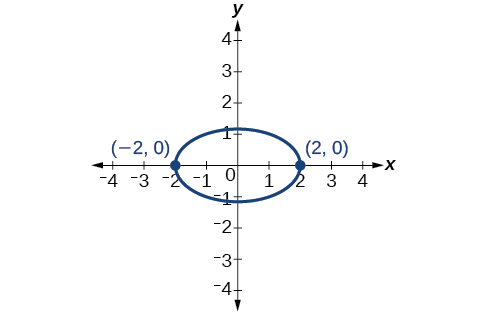$6{x}^{2}+24xy-{y}^{2}-12x+26y+11=0$

## Conic Sections in Polar Coordinates

For the following exercises, given the polar equation of the conic with focus at the origin, identify the eccentricity and directrix.

Hyperbola with $\text{\hspace{0.17em}}e=5\text{\hspace{0.17em}}$ and directrix $\text{\hspace{0.17em}}2\text{\hspace{0.17em}}$ units to the left of the pole.

Ellipse with $\text{\hspace{0.17em}}e=\frac{3}{4}\text{\hspace{0.17em}}$ and directrix $\text{\hspace{0.17em}}\frac{1}{3}\text{\hspace{0.17em}}$ unit above the pole.

For the following exercises, graph the conic given in polar form. If it is a parabola, label the vertex, focus, and directrix. If it is an ellipse or a hyperbola, label the vertices and foci.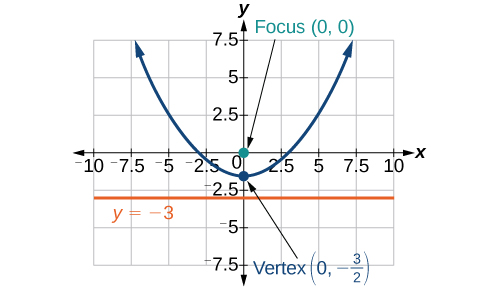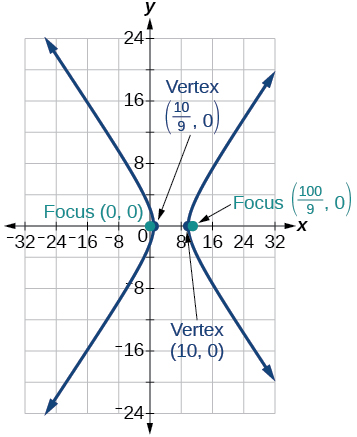For the following exercises, given information about the graph of a conic with focus at the origin, find the equation in polar form.

Directrix is $\text{\hspace{0.17em}}x=3\text{\hspace{0.17em}}$ and eccentricity $\text{\hspace{0.17em}}e=1$

Directrix is $\text{\hspace{0.17em}}y=-2\text{\hspace{0.17em}}$ and eccentricity $\text{\hspace{0.17em}}e=4$

## Practice test

For the following exercises, write the equation in standard form and state the center, vertices, and foci.

$\frac{{x}^{2}}{9}+\frac{{y}^{2}}{4}=1$

$\frac{{x}^{2}}{{3}^{2}}+\frac{{y}^{2}}{{2}^{2}}=1;\text{\hspace{0.17em}}$ center: $\text{\hspace{0.17em}}\left(0,0\right);\text{\hspace{0.17em}}$ vertices: $\text{\hspace{0.17em}}\left(3,0\right),\left(–3,0\right),\left(0,2\right),\left(0,-2\right);\text{\hspace{0.17em}}$ foci: $\left(\sqrt{5},0\right),\left(-\sqrt{5},0\right)$

$9{y}^{2}+16{x}^{2}-36y+32x-92=0$

For the following exercises, sketch the graph, identifying the center, vertices, and foci.

$\frac{{\left(x-3\right)}^{2}}{64}+\frac{{\left(y-2\right)}^{2}}{36}=1$

center: $\text{\hspace{0.17em}}\left(3,2\right);\text{\hspace{0.17em}}$ vertices: $\text{\hspace{0.17em}}\left(11,2\right),\left(-5,2\right),\left(3,8\right),\left(3,-4\right);\text{\hspace{0.17em}}$ foci: $\text{\hspace{0.17em}}\left(3+2\sqrt{7},2\right),\left(3-2\sqrt{7},2\right)$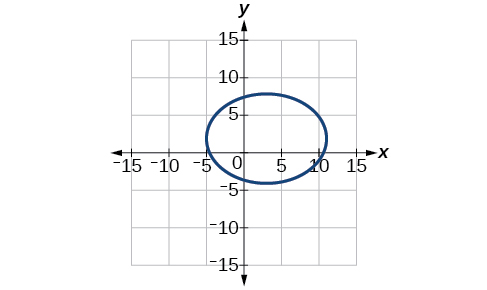$2{x}^{2}+{y}^{2}+8x-6y-7=0$

Write the standard form equation of an ellipse with a center at $\text{\hspace{0.17em}}\left(1,2\right),$ vertex at $\text{\hspace{0.17em}}\left(7,2\right),$ and focus at $\text{\hspace{0.17em}}\left(4,2\right).$

$\frac{{\left(x-1\right)}^{2}}{36}+\frac{{\left(y-2\right)}^{2}}{27}=1$

A whispering gallery is to be constructed with a length of 150 feet. If the foci are to be located 20 feet away from the wall, how high should the ceiling be?

For the following exercises, write the equation of the hyperbola in standard form, and give the center, vertices, foci, and asymptotes.

$\frac{{x}^{2}}{49}-\frac{{y}^{2}}{81}=1$

$\frac{{x}^{2}}{{7}^{2}}-\frac{{y}^{2}}{{9}^{2}}=1;\text{\hspace{0.17em}}$ center: $\text{\hspace{0.17em}}\left(0,0\right);\text{\hspace{0.17em}}$ vertices $\text{\hspace{0.17em}}\left(7,0\right),\left(-7,0\right);\text{\hspace{0.17em}}$ foci: $\text{\hspace{0.17em}}\left(\sqrt{130},0\right),\left(-\sqrt{130},0\right);\text{\hspace{0.17em}}$ asymptotes: $\text{\hspace{0.17em}}y=±\frac{9}{7}x$

$16{y}^{2}-9{x}^{2}+128y+112=0$

For the following exercises, graph the hyperbola, noting its center, vertices, and foci. State the equations of the asymptotes.

$\frac{{\left(x-3\right)}^{2}}{25}-\frac{{\left(y+3\right)}^{2}}{1}=1$

center: $\text{\hspace{0.17em}}\left(3,-3\right);\text{\hspace{0.17em}}$ vertices: $\text{\hspace{0.17em}}\left(8,-3\right),\left(-2,-3\right);$ foci: $\text{\hspace{0.17em}}\left(3+\sqrt{26},-3\right),\left(3-\sqrt{26},-3\right);\text{\hspace{0.17em}}$ asymptotes: $\text{\hspace{0.17em}}y=±\frac{1}{5}\left(x-3\right)-3$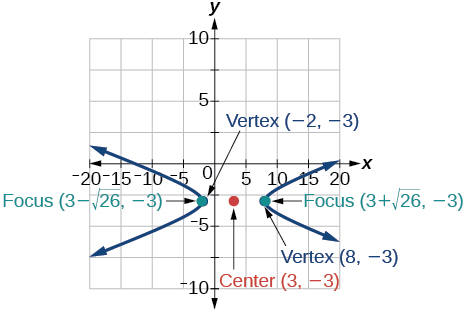${y}^{2}-{x}^{2}+4y-4x-18=0$

Write the standard form equation of a hyperbola with foci at $\text{\hspace{0.17em}}\left(1,0\right)\text{\hspace{0.17em}}$ and $\text{\hspace{0.17em}}\left(1,6\right),$ and a vertex at $\text{\hspace{0.17em}}\left(1,2\right).$

$\frac{{\left(y-3\right)}^{2}}{1}-\frac{{\left(x-1\right)}^{2}}{8}=1$

For the following exercises, write the equation of the parabola in standard form, and give the vertex, focus, and equation of the directrix.

${y}^{2}+10x=0$

$3{x}^{2}-12x-y+11=0$

${\left(x-2\right)}^{2}=\frac{1}{3}\left(y+1\right);\text{\hspace{0.17em}}$ vertex: $\text{\hspace{0.17em}}\left(2,-1\right);\text{\hspace{0.17em}}$ focus: $\text{\hspace{0.17em}}\left(2,-\frac{11}{12}\right);\text{\hspace{0.17em}}$ directrix: $\text{\hspace{0.17em}}y=-\frac{13}{12}$

For the following exercises, graph the parabola, labeling the vertex, focus, and directrix.

${\left(x-1\right)}^{2}=-4\left(y+3\right)$

${y}^{2}+8x-8y+40=0$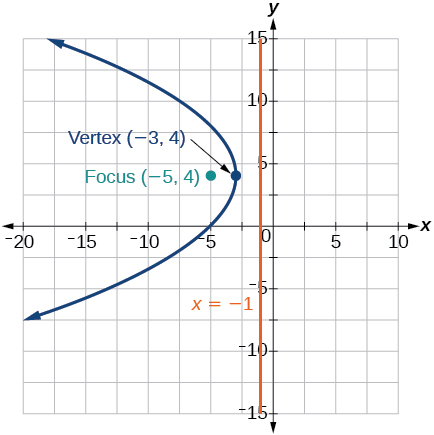Write the equation of a parabola with a focus at $\text{\hspace{0.17em}}\left(2,3\right)\text{\hspace{0.17em}}$ and directrix $\text{\hspace{0.17em}}y=-1.$

A searchlight is shaped like a paraboloid of revolution. If the light source is located 1.5 feet from the base along the axis of symmetry, and the depth of the searchlight is 3 feet, what should the width of the opening be?

Approximately $\text{\hspace{0.17em}}8.49\text{\hspace{0.17em}}$ feet

For the following exercises, determine which conic section is represented by the given equation, and then determine the angle $\text{\hspace{0.17em}}\theta \text{\hspace{0.17em}}$ that will eliminate the $\text{\hspace{0.17em}}xy\text{\hspace{0.17em}}$ term.

$3{x}^{2}-2xy+3{y}^{2}=4$

${x}^{2}+4xy+4{y}^{2}+6x-8y=0$

parabola; $\text{\hspace{0.17em}}\theta \approx {63.4}^{\circ }$

For the following exercises, rewrite in the $\text{\hspace{0.17em}}{x}^{\prime }{y}^{\prime }\text{\hspace{0.17em}}$ system without the $\text{\hspace{0.17em}}{x}^{\prime }{y}^{\prime }\text{\hspace{0.17em}}$ term, and graph the rotated graph.

$11{x}^{2}+10\sqrt{3}xy+{y}^{2}=4$

$16{x}^{2}+24xy+9{y}^{2}-125x=0$

${{x}^{\prime }}^{2}-4{x}^{\prime }+3{y}^{\prime }=0$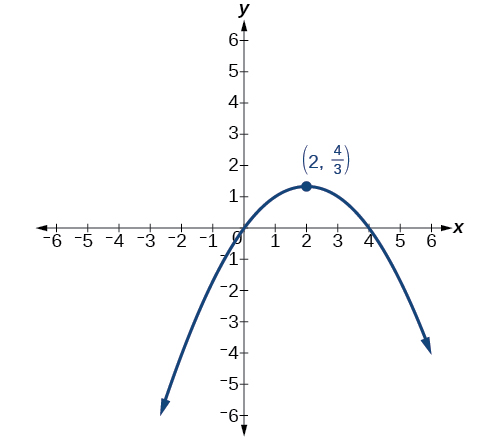For the following exercises, identify the conic with focus at the origin, and then give the directrix and eccentricity.

Hyperbola with $\text{\hspace{0.17em}}e=\frac{3}{2},\text{\hspace{0.17em}}$ and directrix $\text{\hspace{0.17em}}\frac{5}{6}\text{\hspace{0.17em}}$ units to the right of the pole.

For the following exercises, graph the given conic section. If it is a parabola, label vertex, focus, and directrix. If it is an ellipse or a hyperbola, label vertices and foci.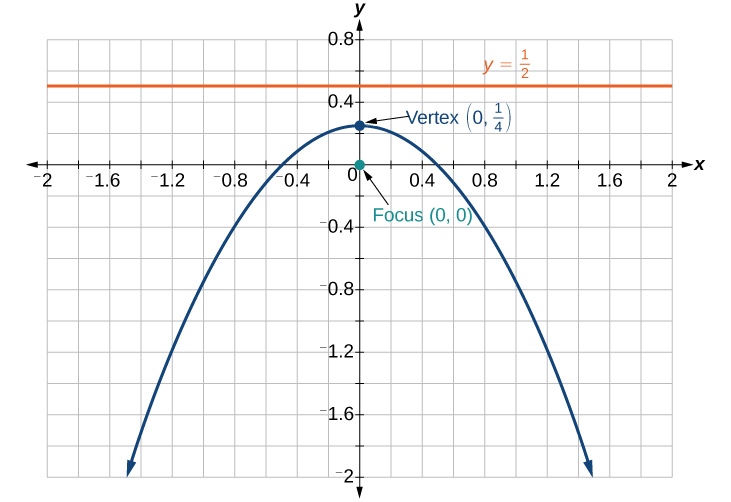Find a polar equation of the conic with focus at the origin, eccentricity of $\text{\hspace{0.17em}}e=2,$ and directrix: $\text{\hspace{0.17em}}x=3.$

#### Questions & Answers

prove that [a+b, b+c, c+a]= 2[a b c]
can't prove
Akugry
i can prove [a+b+b+c+c+a]=2[a+b+c]
this is simple
Akugry
hi
Stormzy
x exposant 4 + 4 x exposant 3 + 8 exposant 2 + 4 x + 1 = 0
x exposent4+4x exposent3+8x exposent2+4x+1=0
HERVE
How can I solve for a domain and a codomains in a given function?
ranges
EDWIN
Thank you I mean range sir.
Oliver
proof for set theory
don't you know?
Inkoom
find to nearest one decimal place of centimeter the length of an arc of circle of radius length 12.5cm and subtending of centeral angle 1.6rad
factoring polynomial
find general solution of the Tanx=-1/root3,secx=2/root3
find general solution of the following equation
Nani
the value of 2 sin square 60 Cos 60
0.75
Lynne
0.75
Inkoom
when can I use sin, cos tan in a giving question
depending on the question
Nicholas
I am a carpenter and I have to cut and assemble a conventional roof line for a new home. The dimensions are: width 30'6" length 40'6". I want a 6 and 12 pitch. The roof is a full hip construction. Give me the L,W and height of rafters for the hip, hip jacks also the length of common jacks.
John
I want to learn the calculations
where can I get indices
I need matrices
Nasasira
hi
Raihany
Hi
Solomon
need help
Raihany
maybe provide us videos
Nasasira
Raihany
Hello
Cromwell
a
Amie
What do you mean by a
Cromwell
nothing. I accidentally press it
Amie
you guys know any app with matrices?
Khay
Ok
Cromwell
Solve the x? x=18+(24-3)=72
x-39=72 x=111
Suraj
Solve the formula for the indicated variable P=b+4a+2c, for b
Need help with this question please
b=-4ac-2c+P
Denisse
b=p-4a-2c
Suddhen
b= p - 4a - 2c
Snr
p=2(2a+C)+b
Suraj
b=p-2(2a+c)
Tapiwa
P=4a+b+2C
COLEMAN
b=P-4a-2c
COLEMAN
like Deadra, show me the step by step order of operation to alive for b
John
A laser rangefinder is locked on a comet approaching Earth. The distance g(x), in kilometers, of the comet after x days, for x in the interval 0 to 30 days, is given by g(x)=250,000csc(π30x). Graph g(x) on the interval [0, 35]. Evaluate g(5)  and interpret the information. What is the minimum distance between the comet and Earth? When does this occur? To which constant in the equation does this correspond? Find and discuss the meaning of any vertical asymptotes.ByByByByBy